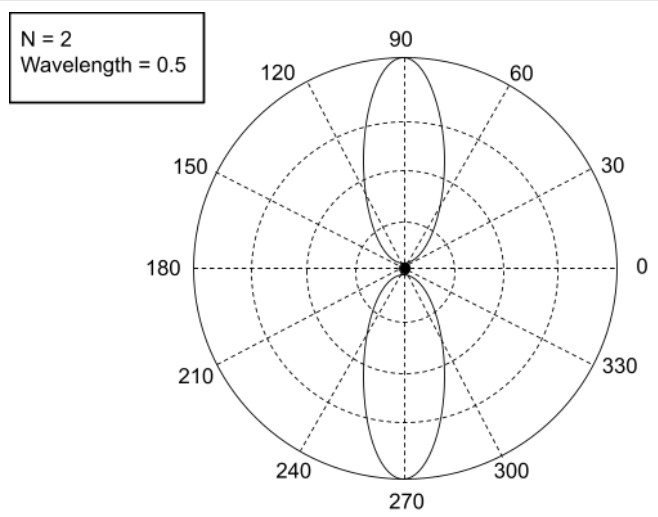# Antenna Array Calculator

Enter the polar angle, wavelength, no. of elements, difference phase between two-element and their distance in the array factor calculator. Click Calculate to find the array factor.

Formula:
Array Factor = sin (N x φ/2)sin(φ/2)

(θ)
(λ)
(β)
N

Give Us Feedback

This phased antenna array calculator can find the overall radiation pattern also known as the array factor of an antenna array. In order to compute the antenna array, place the the required values in given fields.

## What is the Antenna Array factor?

The antenna array is the arrangement of multiple antennas that act like one big antenna.

The overall radiation pattern of an antenna is determined by the array factorIt is represented by AF. By analyzing this factor, the performance of an antenna array can be optimized.

## Antenna array formula

AF = (sin (N x φ/2) / sin(φ/2)

Where the formula to find φ is:

φ = (k x d x cos θ) + β

And for wavelength is:

k = 2 π / λ

In the above equations,

• θ is polar angle
• λ is wavelength
• β is difference between two elements
• N is the number of elements
• d is the distance between elements

## How to find the array factor?

This can be easily understood with the help of an example.

Example

For the given set of data of the antenna array, calculate the array factor.

Polar angle θ = 6

Wavelength λ = 11

The difference between the two elements β  = 13

Number of elements = 2

Distance between elements = 10

Solution:

Step 1: Let's find the wavelength first.

k = π / λ

k = π / 11

K = 0.571

Step 2: Its time to find the value of φ.

φ = (k x d x cos θ) + β

φ = (0.571 x 10 x cos(6)) + 13

φ = 18.68

Step 3: Now find the array factor by putting values in the antenna array formula.

AF = (sin (N x φ/2) / sin(φ/2)

AF = (sin (1 x 18.68/2) / sin(18.68/2)

Array factor = - 1.993

### Math Tools